# Atomic Flashcards

Set Details Share
created 11 years ago by hdiwza
3,126 views
Page to share:
Embed this setcancel
COPY
code changes based on your size selection
Size:
X

1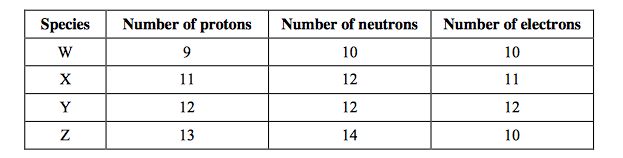Consider the composition of the species W, X, Y and Z below. Which species is an anion?
A. W
B. X
C. Y
D. Z

A

2

Energy levels for an electron in a hydrogen atom are
A. evenly spaced.
B. farther apart near the nucleus.
C. closer together near the nucleus.
D. arranged randomly.

B

3

Which is related to the number of electrons in the outer main energy level of the elements from the alkali metals to the halogens?
I. Group number
II. Period number
A. I only
B. II only
C. Both I and II
D. Neither I nor II

A

4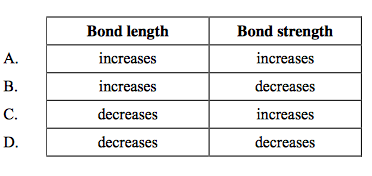How do bond length and bond strength change as the number of bonds between two atoms increases?

C

5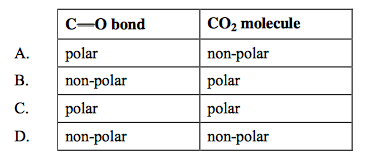Which of the following is true for CO2?

A

6

The molar masses of C2H6, CH3OH and CH3F are very similar. How do their boiling points compare?
A. C2H6 < CH3OH < CH3F
B. CH3F < CH3OH < C2H6
C. CH3OH < CH3F < C2H6
D. C2H6 < CH3F < CH3OH

D

7

What is the electron configuration for an atom with Z = 22?
A. 1s2,2s2,2p6,3s2,3p6,3d4
B. 1s2,2s2,2p6,3s2,3p6,4s2,4p2
C. 1s2,2s2,2p6,3s2,3p6,3d2,4p2
D. 1s2,2s2,2p6,3s2,3p6,4s2,3d2

D

8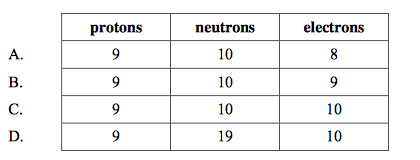What is the correct number of each particle in a fluoride ion, 19F–?

C

9

Which statement is correct for the emission spectrum of the hydrogen atom?
A. The lines converge at lower energies.
B. The lines are produced when electrons move from lower to higher energy levels.
C. The lines in the visible region involve electron transitions into the energy level closest to the nucleus.
D. The line corresponding to the greatest emission of energy is in the ultraviolet region.

D

10

Which is the correct description of polarity in F2 and HF molecules?
A. Both molecules contain a polar bond.
B. Neither molecule contains a polar bond.
C. Both molecules are polar.
D. Only one of the molecules is polar.

D

11

Which types of bonding are present in CH3CHO in the liquid state?
I. Single covalent bonding
II. Double covalent bonding
III. Hydrogen bonding
A. I and II only
B. I and III only
C. II and III only
D. I, II and III

A

12

Which statement(s) is/are generally true about the melting points of substances?
I. Melting points are higher for compounds containing ions than for compounds
containing molecules.
II. A compound with a low melting point is less volatile than a compound with a high melting point.
III. The melting point of a compound is decreased by the presence of impurities.
A. I only
B. I and III only
C. II and III only
D. I, II and III

B

13

What is the correct sequence for the processes occurring in a mass spectrometer?
A. vaporization, ionization, acceleration, deflection
B. vaporization, acceleration, ionization, deflection
C. ionization, vaporization, acceleration, deflection
D. ionization, vaporization, deflection, acceleration

A

14

How many valence electrons are present in an atom of an element with atomic number 16?
A. 2
B. 4
C. 6
D. 8

C

15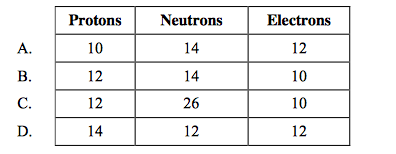How many protons, neutrons and electrons are there in the species 26Mg2+?

B

16

What is the total number of p orbitals containing one or more electrons in germanium (atomic number 32)?
A. 2
B. 3
C. 5
D. 8

D

17

A certain sample of element Z contains 60% of 69Z and 40% of 71Z. What is the relative atomic mass of element Z in this sample?
A. 69.2
B. 69.8
C. 70.0
D. 70.2

B

18

What is the difference between two neutral atoms represented by the symbols 59 Co and 59 Ni?
A. The number of neutrons only.
B. The number of protons and electrons only.
C. The number of protons and neutrons only.
D. The number of protons, neutrons and electrons.

D

19

A certain sample of element Z contains 60% of 69Z and 40% of 71Z. What is the relative atomic mass of element Z in this sample?
A. 69.2
B. 69.8
C. 70.0
D. 70.2

B

20

Which ion would undergo the greatest deflection in a mass spectrometer?
A. 16O+
B. 16O2+
C. 18O2+
D. (16O18O)+

B

21

How many electrons are there in one
A. 10
B. 12
C. 14
D. 22

A

22

The electron arrangement of sodium is 2.8.1. How many occupied main electron energy levels are there in an atom of sodium?
A. 1
B. 3
C. 10
D. 11

B

23

How many electrons are there in all the d orbitals in an atom of xenon?
A. 10
B. 18
C. 20
D. 36

C

24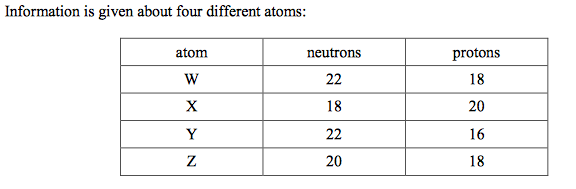Which two atoms are isotopes?
A. W and Y
B. W and Z
C. X and Z
D. Y and Z

B

25

Which statement is correct about a line emission spectrum?
A. Electrons absorb energy as they move from low to high energy levels.
B. Electrons absorb energy as they move from high to low energy levels.
C. Electrons release energy as they move from low to high energy levels.
D. Electrons release energy as they move from high to low energy levels.

D

26

How many neutrons are there in the ion 18O2–?
A. 8
B. 10
C. 16
D. 20

B

27

What is the electron arrangement of silicon?
A. 2.4
B. 2.8
C. 2.8.4
D. 2.8.8

C

28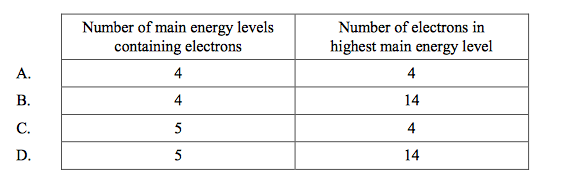Which is correct about the element tin (Sn) (Z = 50)?

C

29

Which statement is correct about the isotopes of an element?
A. They have the same mass number
B. They have the same electron arrangement
C. They have more protons than neutrons
D. They have the same numbers of protons and neutrons

B

30

What is the total number of electrons in p orbitals in an atom of iodine?
A. 5
B. 7
C. 17
D. 23

D

31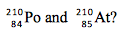What is the difference between two neutral atoms represented by the symbols
A. The number of neutrons only.
B. The number of protons and electrons only.
C. The number of protons and neutrons only.
D. The number of protons, neutrons and electrons.

D

32

Which statements are correct for the emission spectrum of the hydrogen atom?
I. The lines converge at lower energies.
II. Electron transition to n =1 are responsible for lines in the UV region.
III. Lines are produced when electrons move from higher to lower energy levels.
A. I and II only
B. I and III only
C. II and III only
D. I, II and III

C

33

A transition metal ion X2+ has the electronic configuration [Ar]3d9. What is the atomic number of the element?
A. 27
B. 28
C. 29
D. 30

C

34

What is the symbol for a species that contains 15 protons, 16 neutrons and 18 electrons?
D. 31/15 P(3-)

D

35

What is the electron arrangement of an Al3+ ion?
A. 2, 8
B. 2, 3
C. 2, 8, 3
D. 2, 8, 8

A

36

What will happen to the volume of a fixed mass of gas if the pressure and the Kelvin temperature are both doubled?
A. It will remain the same.
B. It will be double its initial volume.
C. It will be one-half its initial volume.
D. It will be four times its initial volume.

A

37

How many orbitals are there in the n = 3 level of an atom?
A. 3
B. 5
C. 7
D. 9

D

38

you can do it...

A

39

What is the correct sequence for the processes occurring in a mass spectrometer?
A. vaporization, ionization, acceleration, deflection
B. vaporization, acceleration, ionization, deflection
C. ionization, vaporization, acceleration, deflection
D. ionization, vaporization, deflection, acceleration

A

40

What is the electron configuration for the copper(I) ion, (Z = 29)?
A. [Ar]4s23d9
B. [Ar]4s13d10
C. [Ar]4s13d9
D. [Ar]3d10

D